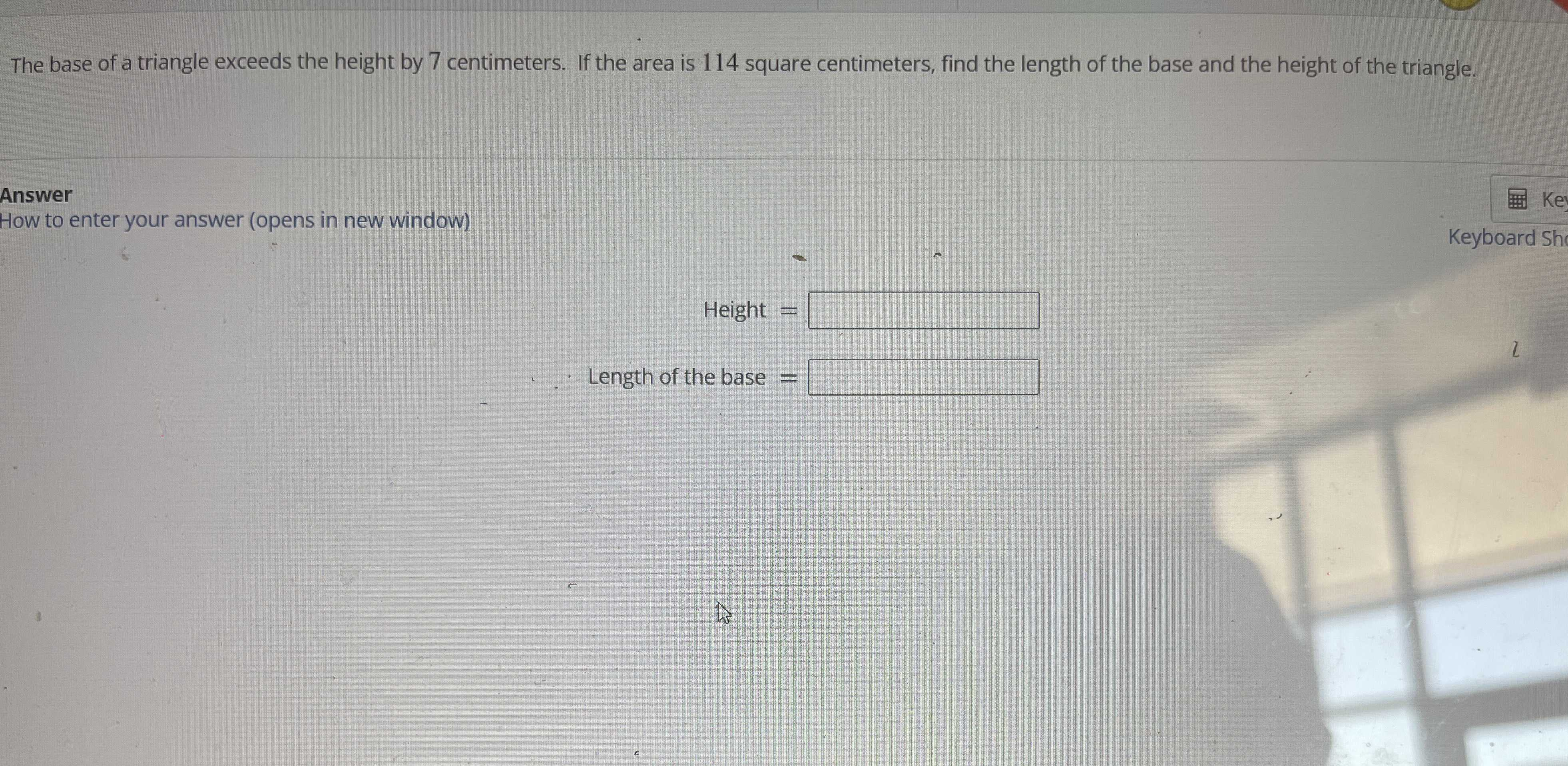### Still have math questions?

Trigonometry
QuestionThe base of a triangle exceeds the height by $$7$$ centimeters. If the area is $$114$$ square centimeters, find the length of the base and the height of the triangle.

height = x

base = x+7

area = 114

114 = $$\frac { 1} { 2}$$×x×(x+7)

x$$^ { 2}$$+7x = 228

x=12

height = 12cm

base = 19cm

Solution
View full explanation on CameraMath App.